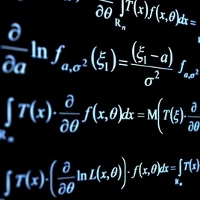by on October 18, 2008

Contributed by Tiberius Lowang

I. Preview

The Dave Male-Female Attraction Equation states that: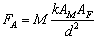where:

• FA is the strength of the mutual attraction between the two people
• AF is the attractiveness of the female
• AM is the attractiveness of the male
• d is the magnitude of the societal pressure to keep the two people apart
• Y is the Yellowness Damping Coefficient

The Yellowness Damping Coefficient is expressed as: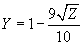where Z represents the fraction of Asianness.

One postulate of Einstein’s Theory of Relativity states that the observed mass of an object increases with speed according to the relation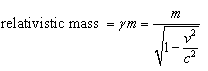where the relativistic mass is the mass of the object moving at a speed v with respect to the observer and m is the mass of the object as measured by an observer at rest with respect to the object. Clearly, the mass of an object increases as its speed increases and approaches the speed of light c.

One thing the Dave Equation cannot explain is why G is a constant in the gravity equation but Y isn’t. This disparity can be explained by the new theory. The relativistic mass equation above can be incorporated into the Dave Equation to expand the original equation into:where:

This theory attributes the variability in Yellowness Damping Coefficient Y to changes in k. For the Asian population, k decreases (dampens) as Z increases. When the male is not Asian, Z equals to 0 and k equals 10. Under the new system, the Mutual Attraction Coefficient M becomes a universal constant analogous to G in Newton’s Law of Universal Gravitation.

kAM is termed relativistic attractiveness of males.

III. Proof

To prove that this equation is not full of shit, here’s the proof that the new equation indeed reduces to Dave’s original equation: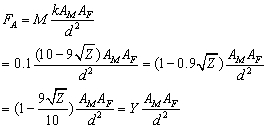Comments on this entry are closed.

Previous post:

Next post: